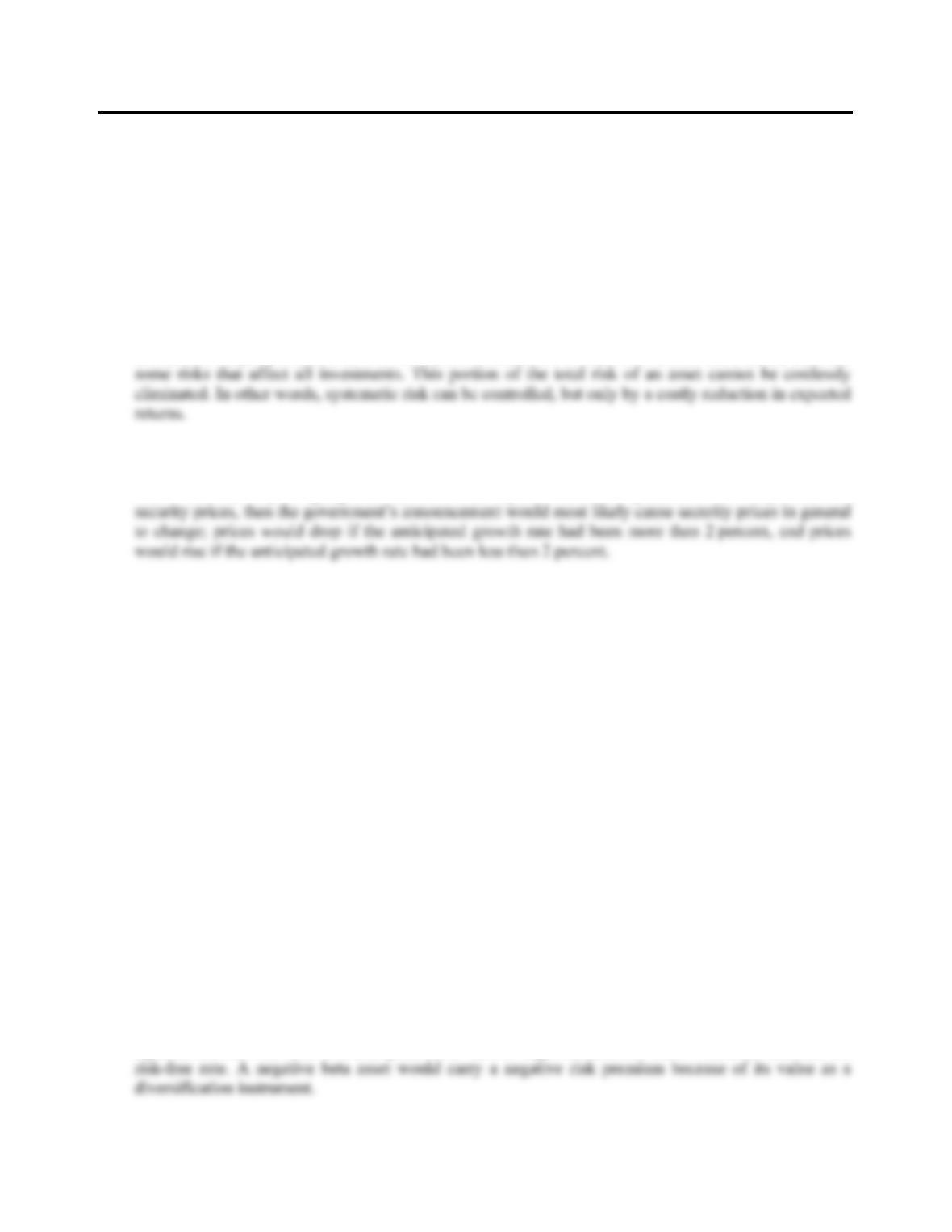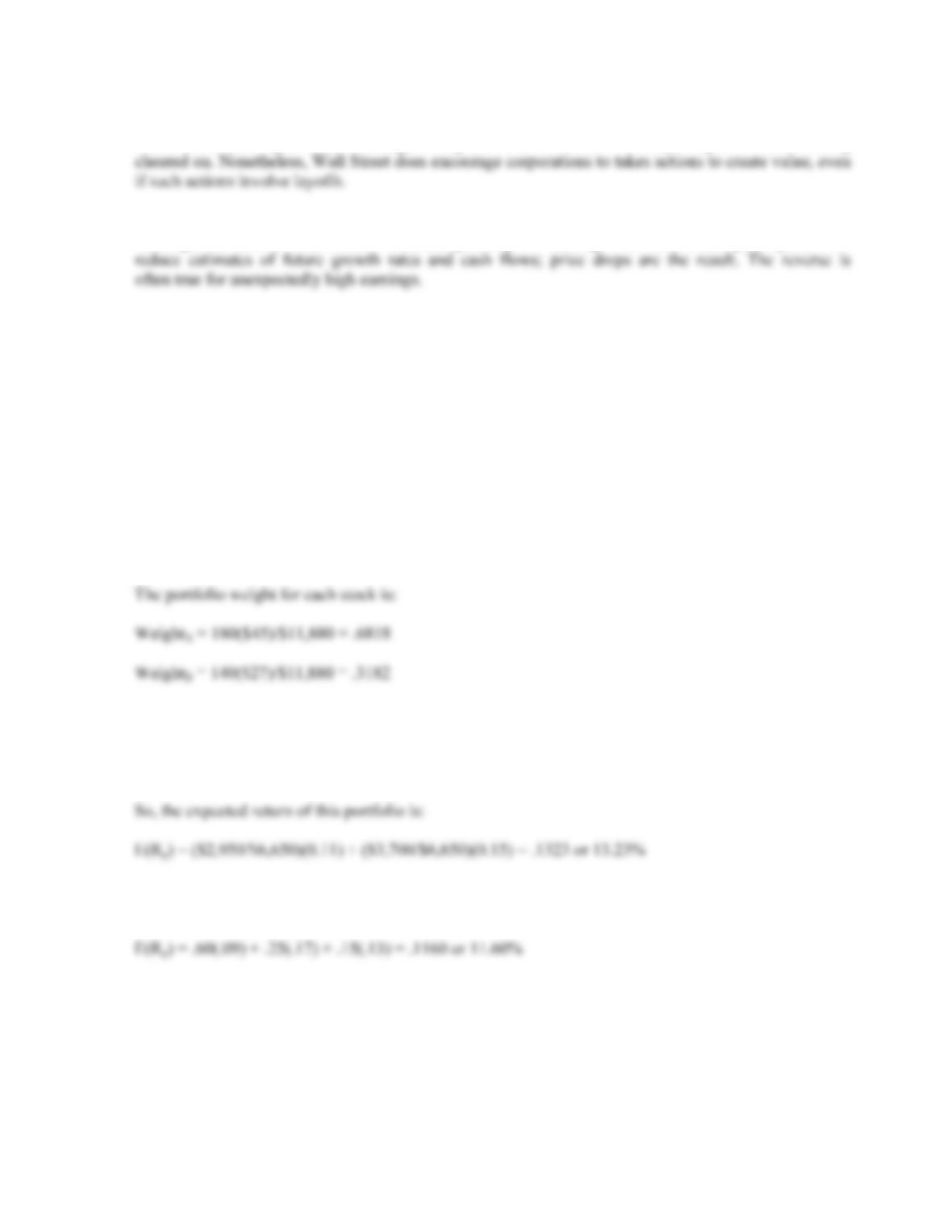Type
Quiz
Book Title
Fundamentals of Corporate Finance Standard Edition 9th Edition
ISBN 13
978-0073382395

### 978-0073382395 Chapter 13 Concepts Review and Critical Thinking Questions

April 3, 2019CHAPTER 13
RISK, RETURN, AND THE SECURITY
MARKET LINE
Answers to Concepts Review and Critical Thinking Questions
1. Some of the risk in holding any asset is unique to the asset in question. By investing in a variety of
assets, this unique portion of the total risk can be eliminated at little cost. On the other hand, there are
2. If the market expected the growth rate in the coming year to be 2 percent, then there would be no
change in security prices if this expectation had been fully anticipated and priced. However, if the
market had been expecting a growth rate other than 2 percent and the expectation was incorporated into
3. a. systematic
b. unsystematic
c. both; probably mostly systematic
d. unsystematic
e. unsystematic
f. systematic
4. a. a change in systematic risk has occurred; market prices in general will most likely decline.
b. no change in unsystematic risk; company price will most likely stay constant.
c. no change in systematic risk; market prices in general will most likely stay constant.
d. a change in unsystematic risk has occurred; company price will most likely decline.
e. no change in systematic risk; market prices in general will most likely stay constant.
5. No to both questions. The portfolio expected return is a weighted average of the asset returns, so it must
be less than the largest asset return and greater than the smallest asset return.
6. False. The variance of the individual assets is a measure of the total risk. The variance on a well-
diversified portfolio is a function of systematic risk only.
7. Yes, the standard deviation can be less than that of every asset in the portfolio. However, p cannot be
less than the smallest beta because p is a weighted average of the individual asset betas.
8. Yes. It is possible, in theory, to construct a zero beta portfolio of risky assets whose return would be
equal to the risk-free rate. It is also possible to have a negative beta; the return would be less than theCHAPTER 13 B-237
9. Such layoffs generally occur in the context of corporate restructurings. To the extent that the market
views a restructuring as value-creating, stock prices will rise. So, it’s not layoffs per se that are being
10. Earnings contain information about recent sales and costs. This information is useful for projecting
future growth rates and cash flows. Thus, unexpectedly low earnings often lead market participants to
Solutions to Questions and Problems
NOTE: All end of chapter problems were solved using a spreadsheet. Many problems require multiple steps.
Due to space and readability constraints, when these intermediate steps are included in this solutions
manual, rounding may appear to have occurred. However, the final answer for each problem is found
without rounding during any step in the problem.
Basic
1. The portfolio weight of an asset is total investment in that asset divided by the total portfolio value.
First, we will find the portfolio value, which is:
Total value = 180(\$45) + 140(\$27) = \$11,880
2. The expected return of a portfolio is the sum of the weight of each asset times the expected return of
each asset. The total value of the portfolio is:
Total value = \$2,950 + 3,700 = \$6,650
3. The expected return of a portfolio is the sum of the weight of each asset times the expected return of
each asset. So, the expected return of the portfolio is: